Isomorphism

(diff) ← Older revision | Latest revision (diff) | Newer revision → (diff)

A correspondence (relation) between objects or systems of objects expressing the equality of their structures in some sense. An isomorphism in an arbitrary category is an invertible morphism, that is, a morphismfor which there exists a morphism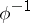such thatand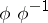are both identity morphisms.

The concept of an isomorphism arose in connection with concrete algebraic systems (initially, with groups) and was extended in a natural way to wider classes of mathematical structures. A classical example of isomorphic, "identically constructed" , systems is the setof real numbers with the operation of addition and the setof positive real numbers with the operation of multiplication.

Letandbe algebraic systems (cf. Algebraic system) of the same type, written in the signature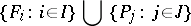with function symbols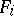,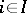, and predicate symbols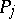,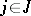: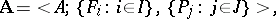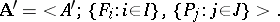An isomorphism, or isomorphic mapping, from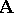ontois a one-to-one mappingfrom the setonto the set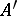with the properties: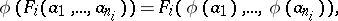for all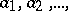inand all,. Thus, in every category of algebraic systems, an isomorphism is a homomorphism that is a bijection. An isomorphism of an algebraic system onto itself is called an automorphism.

The relation of isomorphism is reflexive, symmetric and transitive, that is, it is an equivalence relation splitting any set on which it is defined into disjoint equivalence classes — the classes of pairwise-isomorphic systems. A class of algebraic systems which is a union of such classes is called an abstract class (cf. Algebraic systems, class of).

The isomorphism betweenandmentioned in the main article above can be explicitly given by the means of the exponential mapping or its inverse, the logarithmic function (cf. also Exponential function, real).